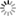##### Personal tools
You are here: Home Mathematics for Machine Learning and Data Science

# Mathematics for Machine Learning and Data Science

### - Overview

Mathematics is the cornerstone of any contemporary scientific discipline. Almost all modern data science techniques, including machine learning, have a deep mathematical foundation.

It goes without saying that you absolutely need all the other intellectual jewels — programming skills, some business acumen, and your unique analytical and curiosity - about data to become a top data scientist.

But it's always worth knowing the mechanics under the hood, rather than just being behind the wheel as someone who knows nothing about cars. So having a solid understanding of the math behind cool algorithms will give you an edge over your peers.

### - Fundamental Math, Tools and Techniques for Data Science

Data science is the process of collecting, analyzing, and modeling data sets. Basic mathematical knowledge is especially important for newcomers entering the field of data science from other industries: hardware engineering, retail, chemical processing industry, medicine and health care, business administration, etc.

While these fields may require experience with spreadsheets, numerical computing and forecasting, the mathematical skills required for data science can vary widely.

Consider a web developer or business analyst. They may process large amounts of data and information on a daily basis, but rigorous modeling of this data may not be emphasized.

Often, the focus is on using the data for immediate needs and moving on, rather than deep scientific exploration. Data science, on the other hand, should always be about science (not data).

Along this line, certain tools and techniques become indispensable. Most are hallmarks of a sound scientific process:

• Model processes (physical or informational) by exploring underlying dynamics
• Build assumptions
• Rigorously estimate the quality of data sources
• Quantifying uncertainty in data and forecasts
• Identify Hidden Patterns from Information Streams
• Understand the limitations of the model
• Understand mathematical proofs and the abstract logic behind them

By its very nature, data science is not restricted to a particular subject area, and may address phenomena as diverse as cancer diagnosis and social behavior analysis.

This yields a dizzying array of possibilities for n-dimensional mathematical objects, statistical distributions, optimization objective functions, and more.### - Mathematics for Machine Learning

Machine learning theory is a field that intersects statistics, probability, computer science, and algorithms to iteratively learn from data and find hidden insights that can be used to build intelligent applications.

Despite the enormous possibilities of machine learning and deep learning, a solid mathematical understanding of many of these techniques is necessary to get a good grasp of the inner workings of algorithms and get good results.

4 Mathematics Pillars that are required for Machine Learning:

• Linear Algebra & Matrix
• Probability & Statistics
• Calculus
• Geometry & Graph Knowledge

### - Mathematics for Data Science

Data science is a broad field that requires a lot of expertise. While math is not the only requirement for a data science career, it is often one of the most important.

Data scientists use math to analyze and understand data. They use mathematical concepts as tools to analyze data and predict results.

Data scientists use three main types of math: Linear algebra, Calculus, Statistics. Data scientists also use probability, which is sometimes grouped together with statistics. Other prerequisites for data science include: Object-oriented programming languages like Java, C, or Python,  Structured Query Language  (SQL) for database queries.

Data science is an interdisciplinary field that uses statistics, scientific computing, and algorithms to extract knowledge and insights from data. It uses techniques and theories from many fields, including mathematics, statistics, computer science, and information science.

[More to come ...]

##### Document Actions